Home > MC2 > Chapter 11 > Lesson 11.3.2 > Problem11-98

11-98.
1. Use the rectangular prism shown below to complete parts (a) through (d) below. Homework Help ✎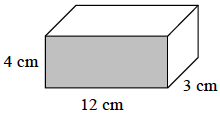1. Calculate the surface area of the prism. Show all your subproblems.

2. Explain why the exponent for area units is 2 (that is, cm2).

3. Calculate the volume of the prism.

4. Explain why the exponent for volume units is a 3 (that is, cm3).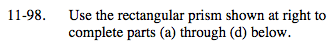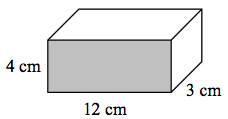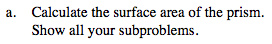To find the surface area, find the area of each face of the prism.

There are 6 faces in a rectangular prism. Each of the three
different faces shown in the image have an opposite face, so find
the area of each of those 3 faces and multiply it by 2.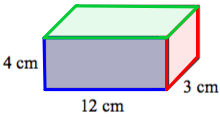$2(4cm)(12cm) + 2(4cm)(3cm) + 2(12cm)(3cm)$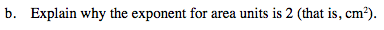How many terms do you multiply together to find area?

To find area, you multiply two dimensions. Just as (x)(x)=x2,
(cm)(cm)=cm2.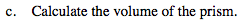To find the volume of a rectangular prism, multiply the base,
height, and width together.

Volume=(12cm)(4cm)(3cm)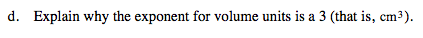See part (b).